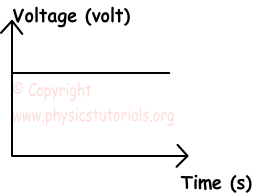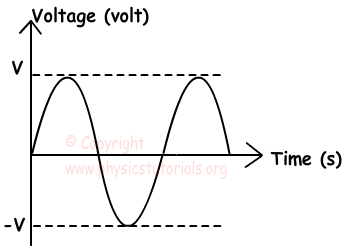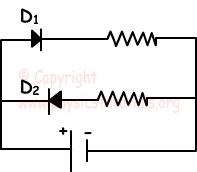Alternating Current and Direct Current and Diodes

Alternating Current and Direct Current

DC means electrons flow only in one direction. Batteries, car batteries are examples of direct current. We analyze the structure of a battery in previous chapters. As you remember, battery has two terminal, “-“and “+”. Electrons are attracted by the “+” terminal and flow of them starts in one direction. In DC, constant voltage supplied to the circuit since the flow is in one direction. Most of the portable devices using battery are work with direct current like CD player, calculator etc.This graph shows the change in the voltage with time. As you can see voltage of the circuit is constant.

On the contrary, AC circuit means that, electrons do not flow in one direction. They move forward and back constantly. As a result of this changing motion of the electrons voltage of the system is also not constant. AC circuits show sinusoidal graphs as given below.Most of the electrical devices in our homes work with alternating current, TV, washing machines, hair drier etc.

You can convert AC to DC with transformer which decreases the amount of voltage, and you can use diodes in your circuits.

Diodes

Diodes are kinds of device that allow current flow only in one direction in circuits. Thus, only half of the cycles of alternating current can pass from the diodes. You can easily convert alternating current into the direct current with this device. Following circuit shows the usage of diodes.In the given circuit, D1 let current flow however, D2 does not let current flow.

Electric Current Exams and Solutions

Author: